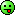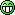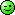# ₦airaland Forum

Welcome, Guest: Join Nairaland / LOGIN! / Trending / Recent / New
Stats: 2,211,245 members, 4,826,975 topics. Date: Thursday, 21 March 2019 at 03:37 PM

## What Is The Total Weights Of The Animals In This Picture? - Education (4) - Nairaland

(1) (2) (3) (4) (Reply) (Go Down)

 Re: What Is The Total Weights Of The Animals In This Picture? by commited(m): 4:40pm On Feb 13, 2015 They will weigh 27kg Re: What Is The Total Weights Of The Animals In This Picture? by Gheebaby(f): 4:50pm On Feb 13, 2015 Yungwizzzy:How are mine supposed to know when it's just a drawing?bring the animals in real life and I'll weigh them simple!!Olodo Re: What Is The Total Weights Of The Animals In This Picture? by chisco82(m): 5:00pm On Feb 13, 2015 Hysmady: You're funny...BtW I love ur package @ ur DPYeye man.Re: What Is The Total Weights Of The Animals In This Picture? by Folksyharry(m): 5:22pm On Feb 13, 2015 husseinjnr:Let A= Rabbit B= Cat C = dogFrom Figure above, in simple analysis A + B = 10kg -----------equation 1A + C = 20kg -----------equation 2B + C = 24 kg ---------- equation 3From equation 2, A= 20- CFrom equation 3B= 24- CSubstitute A and B into equation 120-C + 24-C =1044-2C = 10-2C= 10-44-2C = -34C= 34/2C= 17Substitute C into equation 2A+ 17 = 20A= 3Substitute C into equation 3B+17 = 24B= 7ThereforeA= 3, B= 7, C= 17Total weight is 3+7+17 = 27Rabbit= 3kgCat = 7kgDog = 17kgit won't get any simpler than this Re: What Is The Total Weights Of The Animals In This Picture? by caniva(m): 5:56pm On Feb 13, 2015 It's not 27. you all failed.the correct answer is TWENTY-SEVEN.Lolzzz Re: What Is The Total Weights Of The Animals In This Picture? by FAPatriot(m): 6:11pm On Feb 13, 2015 husseinjnr:Let A= Rabbit B= Cat C = dogFrom Figure above, in simple analysis A + B = 10kg -----------equation 1A + C = 20kg -----------equation 2B + C = 24 kg ---------- equation 3From equation 2, A= 20- CFrom equation 3B= 24- CSubstitute A and B into equation 120-C + 24-C =1044-2C = 10-2C= 10-44-2C = -34C= 34/2C= 17Substitute C into equation 2A+ 17 = 20A= 3Substitute C into equation 3B+17 = 24B= 7ThereforeA= 3, B= 7, C= 17Total weight is 3+7+17 = 27Rabbit= 3kgCat = 7kgDog = 17kgYou must be a mathematics student or teacher. Hanhan.... Re: What Is The Total Weights Of The Animals In This Picture? by ohenhen1: 6:30pm On Feb 13, 2015 27 The dog is 17 The cat is 7 The rabbit is 3Just use deduction, the cat obviously weighs more than the cat. Work from the answers, take 27 and deduce the weight of each animal. Re: What Is The Total Weights Of The Animals In This Picture? by tomzman: 7:54pm On Feb 13, 2015 27kg.I just learnt a shorter method. 1 Like Re: What Is The Total Weights Of The Animals In This Picture? by ify4king(m): 8:28pm On Feb 13, 2015 husseinjnr:Let A= Rabbit B= Cat C = dogFrom Figure above, in simple analysis A + B = 10kg -----------equation 1A + C = 20kg -----------equation 2B + C = 24 kg ---------- equation 3From equation 2, A= 20- CFrom equation 3B= 24- CSubstitute A and B into equation 120-C + 24-C =1044-2C = 10-2C= 10-44-2C = -34C= 34/2C= 17Substitute C into equation 2A+ 17 = 20A= 3Substitute C into equation 3B+17 = 24B= 7ThereforeA= 3, B= 7, C= 17Total weight is 3+7+17 = 27Rabbit= 3kgCat = 7kgDog = 17kgExcellent!!! Re: What Is The Total Weights Of The Animals In This Picture? by ify4king(m): 8:29pm On Feb 13, 2015 lifestyle1: Lets assume rabbit, cat and dog’s weight are R,C and D correspondingly.then we can sayEquation 1: R + C = 10Equation 2: D + R = 20Equation 3: C + D= 24Then we need to find value of C + R + DOn adding Equation 1,2 and 32R + 2 C + 2D = 54 => R+C+D = 27Na expo i use oBig failure Re: What Is The Total Weights Of The Animals In This Picture? by domido(m): 10:06pm On Feb 13, 2015 husseinjnr:Let A= Rabbit B= Cat C = dogFrom Figure above, in simple analysis A + B = 10kg -----------equation 1A + C = 20kg -----------equation 2B + C = 24 kg ---------- equation 3From equation 2, A= 20- CFrom equation 3B= 24- CSubstitute A and B into equation 120-C + 24-C =1044-2C = 10-2C= 10-44-2C = -34C= 34/2C= 17Substitute C into equation 2A+ 17 = 20A= 3Substitute C into equation 3B+17 = 24B= 7ThereforeA= 3, B= 7, C= 17Total weight is 3+7+17 = 27Rabbit= 3kgCat = 7kgDog = 17kgWalahi!!! Bobo yi mowe!!! Omo we ju!!! Efiko! Igi iwe. Ido bale. Owo meji fun eyan kan. Re: What Is The Total Weights Of The Animals In This Picture? by IbnuUche(m): 12:08am On Feb 14, 2015 husseinjnr:Let A= Rabbit B= Cat C = dogFrom Figure above, in simple analysis A + B = 10kg -----------equation 1A + C = 20kg -----------equation 2B + C = 24 kg ---------- equation 3From equation 2, A= 20- CFrom equation 3B= 24- CSubstitute A and B into equation 120-C + 24-C =1044-2C = 10-2C= 10-44-2C = -34C= 34/2C= 17Substitute C into equation 2A+ 17 = 20A= 3Substitute C into equation 3B+17 = 24B= 7ThereforeA= 3, B= 7, C= 17Total weight is 3+7+17 = 27Rabbit= 3kgCat = 7kgDog = 17kg chai mathemathician & their long calculation process. that is why maths scare me at times.very interesting though but i almost confused, i prefer shut cut maths hence i can grab answer & get passing mark. Re: What Is The Total Weights Of The Animals In This Picture? by IbnuUche(m): 12:12am On Feb 14, 2015 Yungwizzzy:How are mine supposed to know when it's just a drawing?bring the animals in real life and I'll weigh them simple!! it seems you hate maths or do you mean practical maths?Re: What Is The Total Weights Of The Animals In This Picture? by IbnuUche(m): 12:26am On Feb 14, 2015 femi4love:27 no giraffing ooohRe: What Is The Total Weights Of The Animals In This Picture? by Nobody: 12:52am On Feb 14, 2015 Yungwizzzy:How are mine supposed to know when it's just a drawing?bring the animals in real life and I'll weigh them simple!!have you seen what is above your post??Yungwizzzy:How are mine supposed to know when it's just a drawing?bring the animals in real life and I'll weigh them simple!!have you seen what is above your post?? Re: What Is The Total Weights Of The Animals In This Picture? by ayobase: 7:22am On Feb 14, 2015 Folksyharry:what will the three animals weigh?here is just a short cut to the stress.Dog appeared twice.Cat appeared twiceRabbit appeared twicetherefore, (10 +20 + 24)/2 = 54/2 = 27. Re: What Is The Total Weights Of The Animals In This Picture? by Folksyharry(m): 8:39am On Feb 14, 2015 ayobase:here is just a short cut to the stress.Dog appeared twice.Cat appeared twiceRabbit appeared twicetherefore, (10 +20 + 24)/2 = 54/2 = 27.that is exactly how I got my answer Re: What Is The Total Weights Of The Animals In This Picture? by femi4love(m): 11:32am On Feb 14, 2015 IbnuUche: no giraffing ooohI actually did it off-head. (No boasting).Re: What Is The Total Weights Of The Animals In This Picture? by SenatorJames(m): 1:14pm On Feb 14, 2015 Answer-27kg.Rat-3kgCat-7kgDog-17kg.Confession: Not by my brain, but by the power and grace to recognize correct answer, and quickly make photocopy. Re: What Is The Total Weights Of The Animals In This Picture? by Vesselofmercy: 11:04pm On Feb 14, 2015 nice brain exercise. ans 27kg; that's rabbit 3kg, cat 7kg and dog 17kg.

(1) (2) (3) (4) (Reply)

(Go Up)

 Sections: politics (1) business autos (1) jobs (1) career education (1) romance computers phones travel sports fashion health religion celebs tv-movies music-radio literature webmasters programming techmarket Nairaland - Copyright © 2005 - 2019 Oluwaseun Osewa. All rights reserved. See How To Advertise. 115Disclaimer: Every Nairaland member is solely responsible for anything that he/she posts or uploads on Nairaland.